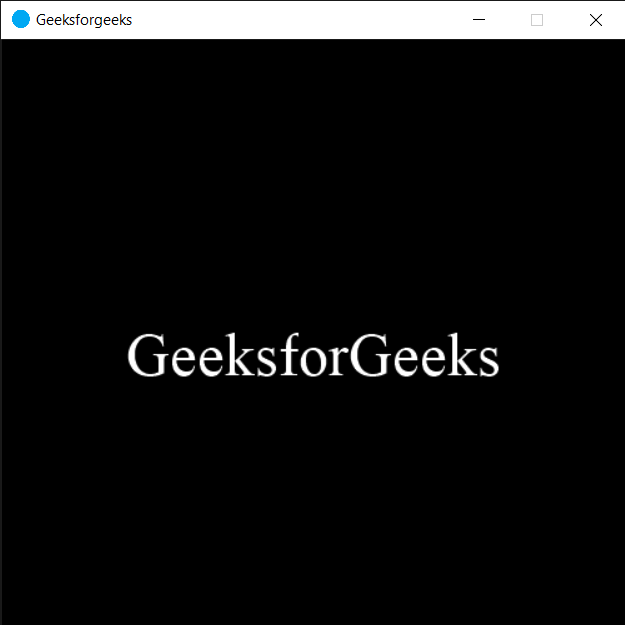Open in App
Not now

# PYGLET – Getting Window Screen

• Last Updated : 22 Oct, 2021

In this article we will see how we can get the window resizable property in PYGLET module in python. Pyglet is easy to use but powerful library for developing visually rich GUI applications like games, multimedia etc. A window is a “heavyweight” object occupying operating system resources. Windows may appear as floating regions or can be set to fill an entire screen (fullscreen). Screen object is the screen this window is fullscreen in, used by the window to display the content.
We can create a window with the help of command given below

` pyglet.window.Window(width, height, title)`

In order to create window we use screen attribute with the window object
Syntax : window.screen
Argument : It takes no argument
Return : It returns Win32Screen object

Below is the implementation

## Python3

 `# importing pyglet module``import` `pyglet``import` `pyglet.window.key` `# width of window``width ``=` `500` `# height of window``height ``=` `500` `# caption i.e title of the window``title ``=` `"Geeksforgeeks"` `# creating a window``window ``=` `pyglet.window.Window(width, height, title)` `# text``text ``=` `"GeeksforGeeks"` `# creating a label with font = times roman``# font size = 36``# aligning it to the centre``label ``=` `pyglet.text.Label(text,``                          ``font_name ``=``'Times New Roman'``,``                          ``font_size ``=` `36``,``                          ``x ``=` `window.width``/``/``2``, y ``=` `window.height``/``/``2``,``                          ``anchor_x ``=``'center'``, anchor_y ``=``'center'``)` `new_label ``=` `pyglet.text.Label(text,``                          ``font_name ``=``'Times New Roman'``,``                          ``font_size ``=` `10``,``                          ``x ``=` `25``, y ``=` `25``)` `# on draw event``@window``.event``def` `on_draw():   ` `    ` `    ``# clearing the window``    ``window.clear()``    ` `    ``# drawing the label on the window``    ``label.draw()` `    ` `# key press event   ``@window``.event``def` `on_key_press(symbol, modifier):` `    ``# key "C" get press``    ``if` `symbol ``=``=` `pyglet.window.key.C:``        ` `        ``# closing the window``        ``window.close()``    `  `# image for icon``img ``=` `image ``=` `pyglet.resource.image(``"logo.png"``)` `# setting image as icon``window.set_icon(img)` `# getting window screen``value ``=` `window.screen` `# printing the value``print``(``"Window Screen : "``)``print``(value)` `               ` `# start running the application``pyglet.app.run()`

Output :```Window resizable ? :
Win32Screen(x=0, y=0, width=1536, height=864)```

My Personal Notes arrow_drop_up